# Verification of Ohm's Law using Voltmeter and Ammeter

## Problems from IIT JEE

Problem (IIT JEE 2004): Draw the circuit for experimental verification of Ohm's law using a source of variable DC voltage, a main resistance of $100\; \Omega$, two galvanometers and two resistances of values $10^6\; \Omega$ and $10^-3\;\Omega$, respectively. Clearly show the positions of the voltmeter and the ammeter.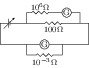The Ohm's law verification requires measurement of the voltage and current. The galvanometer can be converted to a voltmeter by connecting very high resistance (${10}^{6}\;\Omega$) in series and to an ammeter by connecting very low resistance ${10}^{-3}\; \Omega$) in parallel, as shown.

Problem (IIT JEE 2010): To verify Ohm's law, a student is provided with a test resister $R_T$, a high resistance $R_1$, and a small resistance $R_2$, two identical galvanometers $G_1$ and $G_2$, and a variable voltage source $V$. The correct circuit to carry out the experiment is,

1.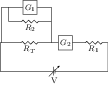2.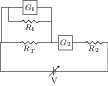3.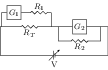4.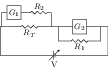Solution: To verify Ohm's law, we need to measure voltage across the test resistance $R_T$ and current passing through it. The voltage can be measured by connecting high resistance $R_1$ in series with galvanometer. This combination becomes a voltmeter and shall be connected in parallel to $R_T$. The current can be measured by connecting low resistance $R_2$ in parallel with galvanometer. This combination becomes an ammeter and shall be connected in series to measure current through $R_T$. Hence answer is C.